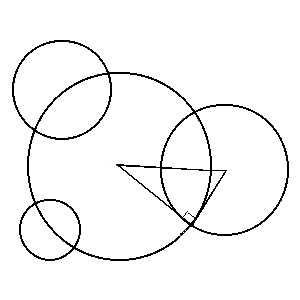#### You may also like### Baby Circle

A small circle fits between two touching circles so that all three circles touch each other and have a common tangent? What is the exact radius of the smallest circle?### Kissing

Two perpendicular lines are tangential to two identical circles that touch. What is the largest circle that can be placed in between the two lines and the two circles and how would you construct it?### Logosquares

Ten squares form regular rings either with adjacent or opposite vertices touching. Calculate the inner and outer radii of the rings that surround the squares.

# Orthogonal Circle

##### Age 16 to 18 Challenge Level:

Congratulations to Tony from State College Area High School, Pennsylvania, USA for this solution.

First of all, here is the solution to finding the equation of the orthogonal circle for the circles with centers of $(0,0)$, $(3,0)$, $(9,2)$ and radii respectively of $5$, $4$, and $6$.

As the circles are orthogonal we can draw three right angled triangles. One of the legs of each right triangle is the radius of one of the given circles, the other leg is the radius of the unknown orthogonal circle, and the hypotenuse is the distance between the center of the known circle and the center of the unknown orthogonal circle.Since we have three given circles, we can use the Pythagorean Theorem three times to find our answer. Here are the equations that find the center (h,k) of the orthogonal circle with radius r:

$\begin {eqnarray} 5^2 + r^2 &= h^2 + k^2 \quad (A) \\ 4^2 + r^2 &= (h-3)^2 + k^2 \quad (B) \\ 6^2 + r^2 &= (h-9)^2 + (k-2)^2 \quad (C) \\ \end {eqnarray}$

Now subtract equation (B) from equation (A) to get $18 = 6h$, so $h=3$. Now plug $h=3$ in equation (A) to get $r^2 + 16 = k^2.$ Next plug $h=3$ and $k^2 = r^2 + 16$ into equation (C) to get :

$r^2 + 36 = (-6)^2 + (r^2 + 16) -4k + 4$

from which we find $k = 5.$ Plug $k=5$ in equation (A) to get $r=3.$ So now we have all we need to make the equation of the orthogonal circle: $h=3$, $k=5$ and $r=3$, so the equation of the orthogonal circle is $(x-3)^2 + (y-5)^2=9.$

When we try to find the orthogonal circle for the second example, we end up with simultaneous equations which are fruitless, unsolvable. This means the second equation has an orthogonal line, in this case a fairly obviously one with $y=x.$

Now I will prove that you have an orthogonal line when the centers of the circles are collinear, otherwise you have an orthogonal circle.

Any orthogonal line would have to form a right angle with every given circle. When a line forms a right angle with the circumference of the circle, that means it passes through the center of the circle, so our orthogonal line passes through the centers of all the circles. The only way this is possible for our line to accomplish this (since two points determine a line, three points determine a plane), is if all three centers are collinear.

Therefore, lines can handle every case of collinear centers, and since an orthogonal line or circle can always be drawn, every other case is covered by an orthogonal circle.

[We have used Tony's American spelling of center here. Ed.]